Square Roots 5
Set 5 (10 Questions)
Students use exponents, powers, and roots, and use exponents in working with fractions
From Mr. Anker Tests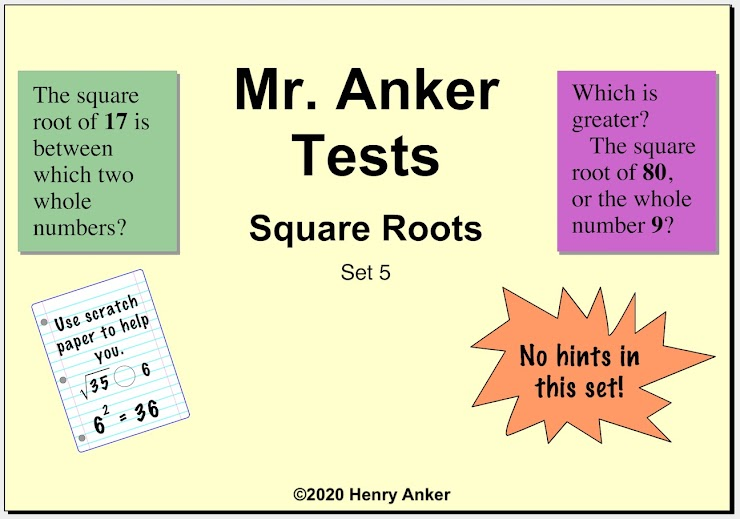Square Roots Help Slide 1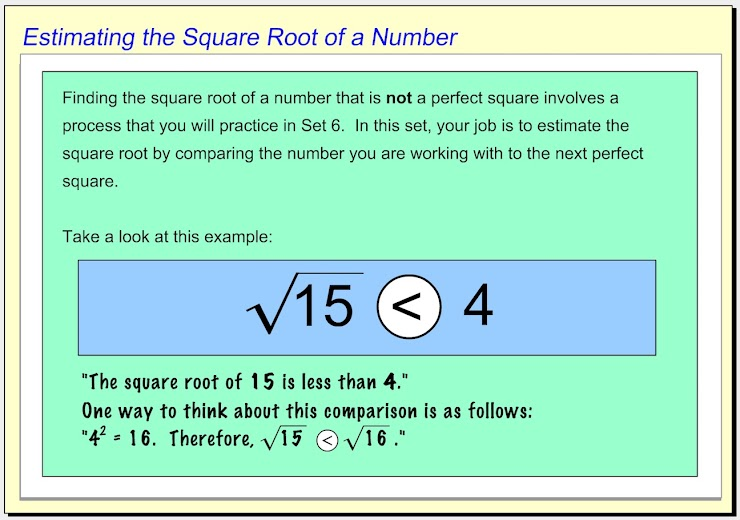Square Roots Help Slide 2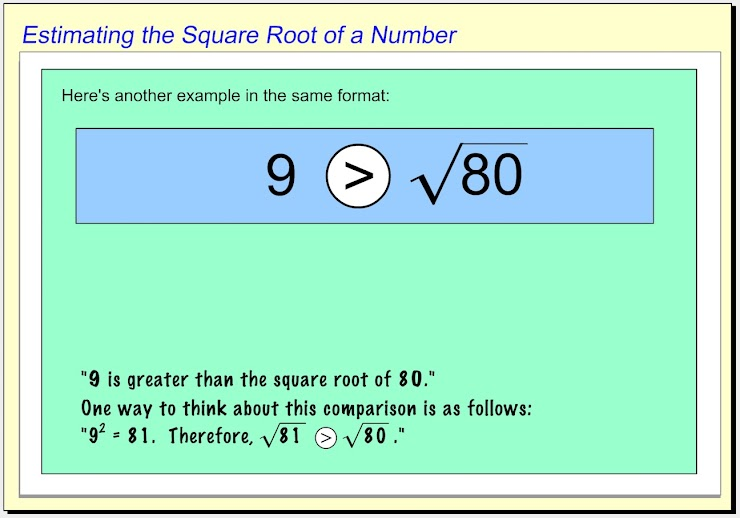Square Roots Help Slide 3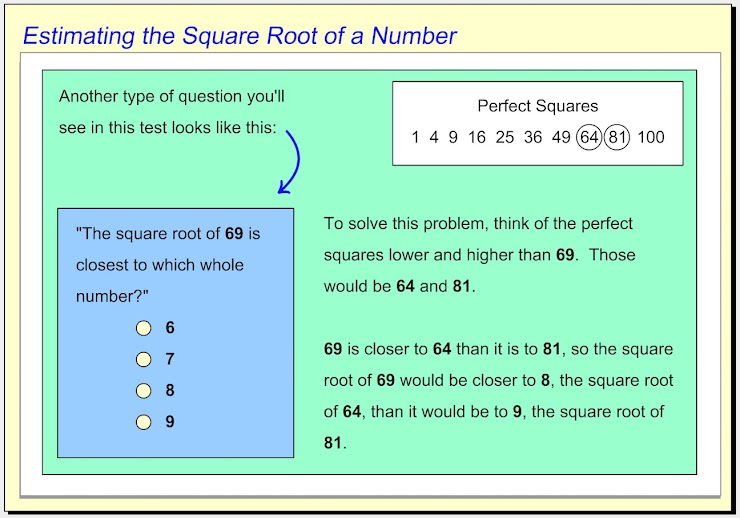Square Roots Help Slide 4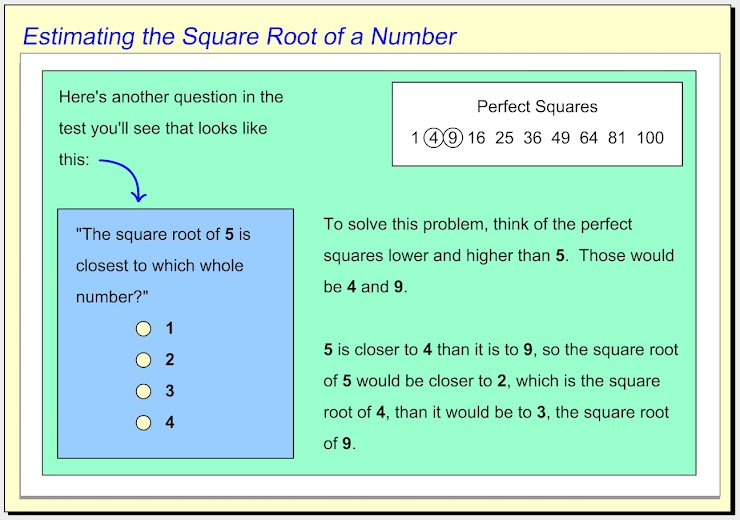1.  The square root of  15  is between which two whole numbers? *
1 point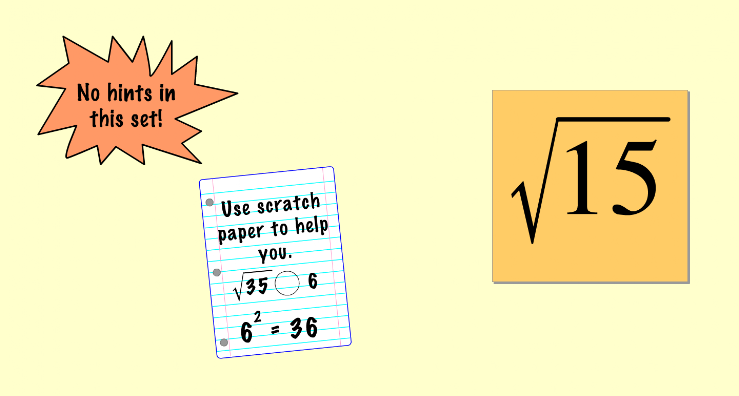2.  The square root of  99  is between which two whole numbers? *
1 point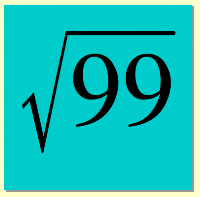3. Use the dropdown menu below to make this statement true: "Five is __________ the square root of twenty-two." *
1 point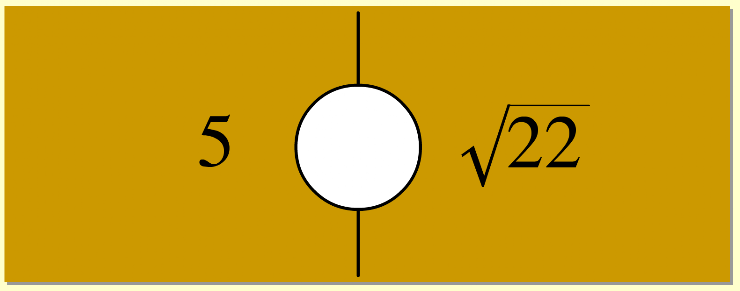4. Use the dropdown menu below to make this statement true: "The square root of thirty-seven is __________ six." *
1 point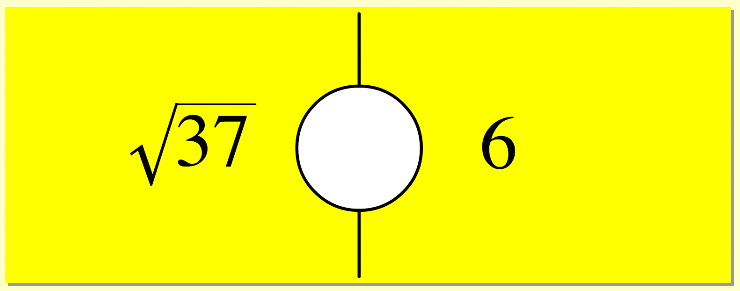5.  The square root of  140  is closest to which whole number? *
1 point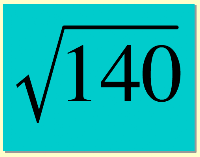6.  Use the dropdown menu below to make this statement true: "The square root of seventy is __________ eight." *
1 point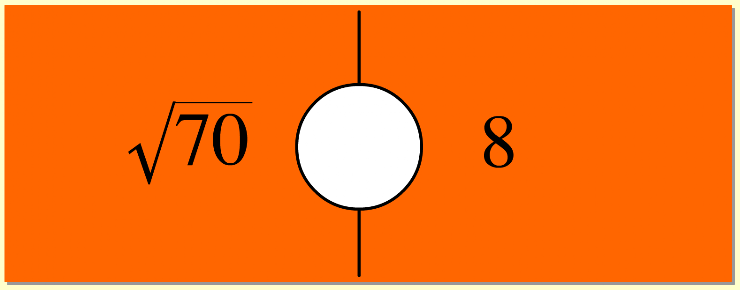7.  The square root of  120  is closest to which whole number? *
1 point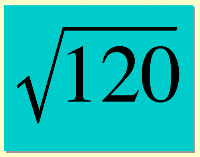8.  Use the dropdown menu below to make this statement true: "The square root of sixty-four is __________ eight." *
1 point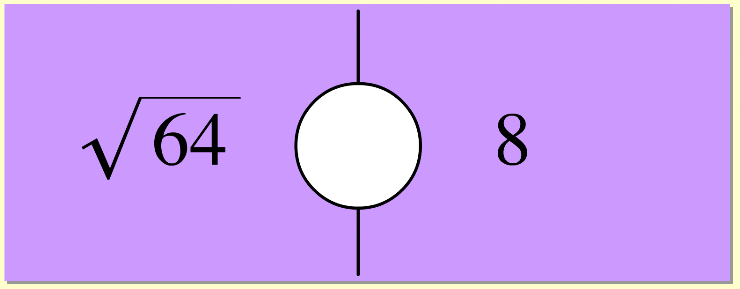9.  Use the dropdown menu below to make this statement true: "The square root of one hundred twenty-five is __________ twenty-five." *
1 point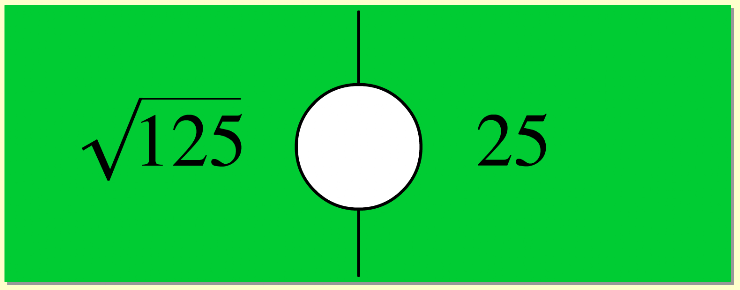10.  Use the dropdown menu below to make this statement true: "The square root of one hundred five is __________ eleven." *
1 point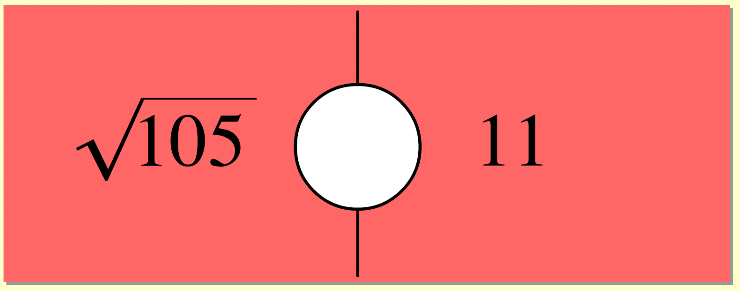Submit
Clear form